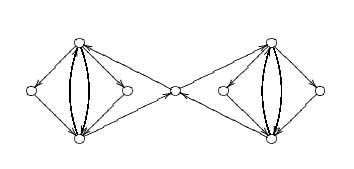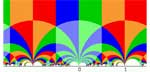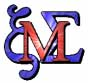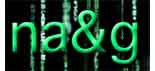Tag: Cuntz

Now that the preparation for my undergraduate courses in the first semester is more or less finished, I can begin to think about the courses I’ll give this year in the master class
non-commutative geometry. For a change I’d like to introduce the main ideas and concepts by a very concrete example : Ginzburg’s coadjoint-orbit result for the Calogero-Moser space and its
relation to the classification of one-sided ideals in the first Weyl algebra. Not only will this example give me the opportunity to say things about formally smooth algebras, non-commutative
differential forms and even non-commutative symplectic geometry, but it also involves what some people prefer to call _non-commutative algebraic geometry_ (that is the study of graded Noetherian
rings having excellent homological properties) via the projective space associated to the homogenized Weyl algebra. Besides, I have some affinity with this example.

A long time ago I introduced
the moduli spaces for one-sided ideals in the Weyl algebra in Moduli spaces for right ideals of the Weyl algebra and when I was printing a _very_ preliminary version of Ginzburg’s paper
Non-commutative Symplectic Geometry, Quiver varieties, and Operads (probably because he send a preview to Yuri Berest and I was in contact with him at the time about the moduli spaces) the
idea hit me at the printer that the right way to look at the propblem was to consider the quiver

$\xymatrix{\vtx{} \ar@/^/[rr]^a & & \vtx{} \ar@(u,ur)^x \ar@(d,dr)_y \ar@/^/[ll]^b}$

which eventually led to my paper together with Raf Bocklandt Necklace Lie algebras and noncommutative symplectic geometry.

Apart from this papers I would like to explain the following
papers by illustrating them on the above example : Michail Kapranov Noncommutative geometry based on commutator expansions Maxim Kontsevich and Alex Rosenberg Noncommutative smooth
spaces
Yuri Berest and George Wilson Automorphisms and Ideals of the Weyl Algebra Yuri Berest and George Wilson Ideal Classes of the Weyl Algebra and Noncommutative Projective
Geometry
Travis Schedler A Hopf algebra quantizing a necklace Lie algebra canonically associated to a quiver and of course the seminal paper by Joachim Cuntz and Daniel Quillen on
quasi-free algebras and their non-commutative differential forms which, unfortunately, in not available online.

I plan to write a series of posts here on all this material but I will be very
your name and email is required to do so, you do not have to register and you can even put some latex-code in your post but such a posting will first have to viewed by me to avoid cluttering of
nonsense GIFs in my directories).Before the vacation I finished a rewrite of the One quiver to rule them
all
note. The main point of that note was to associate to any qurve
$A$ (formerly known as a quasi-free algebra in the terminology of
Cuntz-Quillen or a formally smooth algebra in the terminology of
Kontsevich-Rosenberg) a quiver $Q(A)$ and a dimension vector $\alpha_A$
such that $A$ is etale isomorphic (in a yet to be defined
non-commutative etale toplogy) to a ring Morita equivalent to the path
algebra $lQ(A)$ where the Morita setting is determined by the dimension
vector $\alpha_A$. These “one-quiver settings” are easy to
work out for a group algebra $lG$ if $G$ is the amalgamated free product
of finite groups $G = H_1 \bigstar_H H_2$.

Here is how to do
this : construct a bipartite quiver with the left vertices corresponding
to the irreducible representations of $H_1$, say ${ S_1, .. ,S_k }$ of
dimensions $(d_1, .. ,d_k)$ and the right vertices corresponding to the
irreducible representations of $H_2$, ${ T_1, .. ,T_l }$ of dimensions
$(e_1, .. ,e_l)$. The number of arrows from the $i$-th left vertex to
the $j$-th right vertex is given by the dimension of $Hom_H(S_i,T_j)$
This is the quiver I call the Zariski quiver for $G$ as the finite
dimensional $G$-representations correspond to $\theta$-semistable
representations of this quiver for the stability structure $\theta=(d_1, .. ,d_k ; -e_1, .. ,-e_l)$. The one-quiver $Q(G)$ has vertices
corresponding to the minimal $\theta$-stable dimension vectors (say
$\alpha,\beta, ..$of the Zariski quiver and with the number of arrows
between two such vertices determined by $\delta_{\alpha \beta}-\chi(\alpha,\beta)$ where $\chi$ is the Euler form of the Zariski
quiver. In the old note I've included the example of the projective
modular group $PSL_2(Z) = Z_2 \bigstar Z_3$ (which can easily be
generalized to the modular group $SL_2(Z) = Z_4 \bigstar_{Z_2} Z_6$)
which turns out to be the double of the extended Dynkin quiver
$\tilde{A_5}$. In the rewrite I've also included an example of a
congruence subgroup $\Gamma_0(2) = Z_4 \bigstar_{Z_2}^{HNN}$ which is an
HNN-extension. These are somehow the classical examples of interesting
amalgamated (HNN) groups and one would like to have plenty of other
interesting examples. Yesterday I read a paper by Karen Vogtmann called

Automorphisms of free groups and outer space
in which I encountered
an amalgamated product decomposition for $GL_2(Z) = D_8 \bigstar_{Z_2 \times Z_2} (S_3 \times Z_2)$where $D_8$ is the diheder group of 8
elements. When I got back from vacation I found a reference to this
result in my mail-box from Warren Dicks. Theorem 23.1, p. 82, in Heiner
Zieschang, Finite Groups of Mapping Classes of Surfaces, LNM 875,
Springer, Berlin, 1981.

I worked out the one-quiver and it has
the somewhat strange form depicted above. It is perfectly possible that
I made mistakes so if you find another result, please let me know.

the correct one quiver can be found elsewhere on this blog.Can
it be that one forgets an entire proof because the result doesn’t seem
important or relevant at the time? It seems the only logical explanation
for what happened last week. Raf Bocklandt asked me whether a
classification was known of all group algebras l G which are
noncommutative manifolds (that is, which are formally smooth a la Kontsevich-Rosenberg or, equivalently, quasi-free
a la Cuntz-Quillen). I said I didn’t know the answer and that it looked
like a difficult problem but at the same time it was entirely clear to
me how to attack this problem, even which book I needed to have a look
at to get started. And, indeed, after a visit to the library borrowing
Warren Dicks
lecture notes in mathematics 790 “Groups, trees and projective
modules” and browsing through it for a few minutes I had the rough
outline of the classification. As the proof is basicly a two-liner I
might as well sketch it here.
If l G is quasi-free it
must be hereditary so the augmentation ideal must be a projective
module. But Martin Dunwoody proved that this is equivalent to
G being a group acting on a (usually infinite) tree with finite
group vertex-stabilizers all of its orders being invertible in the
basefield l. Hence, by Bass-Serre theory G is the
fundamental group of a graph of finite groups (all orders being units in
l) and using this structural result it is then not difficult to
show that the group algebra l G does indeed have the lifting
property for morphisms modulo nilpotent ideals and hence is
quasi-free.
If l has characteristic zero (hence the
extra order conditions are void) one can invoke a result of Karrass
saying that quasi-freeness of l G is equivalent to G being
virtually free (that is, G has a free subgroup of finite
index). There are many interesting examples of virtually free groups.
One source are the discrete subgroups commensurable with SL(2,Z)
(among which all groups appearing in monstrous moonshine), another
source comes from the classification of rank two vectorbundles over
projective smooth curves over finite fields (see the later chapters of
Serre’s Trees). So
one can use non-commutative geometry to study the finite dimensional
representations of virtually free groups generalizing the approach with
Jan Adriaenssens in Non-commutative covers and the modular group (btw.
Jan claims that a revision of this paper will be available soon).
In order to avoid that I forget all of this once again, I’ve
written over the last couple of days a short note explaining what I know
of representations of virtually free groups (or more generally of
fundamental algebras of finite graphs of separable
l-algebras). I may (or may not) post this note on the arXiv in
the coming weeks. But, if you have a reason to be interested in this,
send me an email and I’ll send you a sneak preview.Bill
Schelter was a remarkable man. First, he was a top-class mathematician.
If you allow yourself to be impressed, read his proof of the
Artin-Procesi theorem. Bill was also among the first to take
non-commutative geometry seriously. Together with Mike Artin he
investigated a notion of non-commutative integral extensions and he was
the first to focuss attention to formally smooth algebras (a
suggestion later taken up by a.o. Cuntz-Quillen and Kontsevich) and a
relative version with respect to algebras satisfying all identities of
n x n matrices which (via work of Procesi) led to smooth@n
algebras. To youngsters, he is probably best know as the co-inventor of
Artin-Schelter regular algebras. I still vividly remember an
overly enthusiastic talk by him on the subject in Oberwolfach, sometime
in the late eighties. Secondly, Bill was a genuine Lisp-guru and
a strong proponent of open source software, see for example his
petition against software patents. He maintanind
his own version of Kyoto Common Lisp which developed into Gnu
Common Lisp
. A quote on its history :

GCL is
the product of many hands over many years. The original effort was known
as the Kyoto Common Lisp system, written by Taiichi Yuasa and Masami
Hagiya in 1984. In 1987 new work was begun by William Schelter, and that
version of the system was called AKCL (Austin Kyoto Common Lisp). In
1994 AKCL was released as GCL (GNU Common Lisp) under the GNU public
library license. The primary purpose of GCL during that phase of it’s
existence was to support the Maxima computer algebra system, also
maintained by Dr. Schelter. It existed largely as a subproject of
Maxima.

Maxima started as Bill’s version of
Macsyma an MIT-based symbolic computation program to which he
added many routines, one of which was Affine a package that
allowed to do Groebner-like computations in non-commutative
algebras (implementing Bergman’s diamond lemma) and which he
needed to get a grip on 3-dimensional Artin-Schelter regular
algebras
. Michel and me convinced Fred to acquire funds to
buy us a work-station (costing at the time 20 to 30 iMacs) and have Bill
flown in from the States with his tape of maxima and let him
port it to our Dec-station. Antwerp was probably for years
the only place in the world (apart from MIT) where one could do
calculations in affine (probably highly illegal at the time).
Still, lots of people benefitted from this, among others Michaela
Vancliff
and Kristel Van Rompay in their investigation
of 4-dimensional Artin-Schelter regular algebras associated to an
automorphism of a quadric in three-dimensional projective space.
Yesterday I ran into Bill (alas virtually) by browsing the
crypto-category of Fink. There it was, maxima, Bill’s package! I tried to install it
with the Fink Commander and failed but succeeded from the command line.
So, if you want to have your own version of it type

sudo fink
install maxima

from the Terminal and it will install without
problems (giving you also a working copy of common lisp). Unfortunately
I do not remember too much of Macsyma or Affine but there is plenty of
documentation on the net. Manuals and user guides can be obtained from
the maxima homepage and the University of Texas
(Bill’s university) maintains an online manual, including a cryptic description of
some Affine-commands. But probably I’ll have to send Michaela an
email asking for some guidance on this… Here, as a tribute to Bill who
died in july 2001 the opening banner

 iMacLieven:~ lieven\$
/sw/bin/maxima Maxima 5.9.0 http://maxima.sourceforge.net
See the file COPYING.
Dedicated to the memory of William Schelter.
This is a development version of Maxima.
The function bug_report() provides bug reporting information.
(C1)Yesterday I made reservations for lecture rooms to run the
master class on non-commutative geometry sponsored by the ESF-NOG project. We have a lecture room on
monday- and wednesday afternoon and friday the whole day which should be
enough. I will run two courses in the program : non-commutative
geometry
and projects in non-commutative geometry both 30
hours. I hope that Raf Bocklandt will do most of the work on the
Geometric invariant theory course so that my contribution to it
can be minimal. Here are the first ideas of topics I want to cover in my
courses. As always, all suggestions are wellcome (just add a
comment).

non-commutative geometry : As
I am running this course jointly with Markus Reineke and as Markus will give a
mini-course on his work on non-commutative Hilbert schemes, I will explain
the theory of formally smooth algebras. I will cover most of the
paper by Joachim Cuntz and Daniel Quillen “Algebra extensions and
nonsingularity”, Journal of AMS, v.8, no. 2, 1995, 251?289. Further,
I’ll do the first section of the paper by Alexander Rosenberg and Maxim Kontsevich,
Noncommutative smooth spaces“. Then, I will
explain some of my own work including the “One
quiver to rule them all
” paper and my recent attempts to classify
all formally smooth algebras up to non-commutative birational
equivalence. When dealing with the last topic I will explain some of Aidan Schofield‘s paper
Birational classification of moduli spaces of representations of quivers“.

projects in
non-commutative geometry
: This is one of the two courses (the other
being “projects in non-commutative algebra” run by Fred Van Oystaeyen)
for which the students have to write a paper so I will take as the topic
of my talks the application of non-commutative geometry (in particular
the theory of orders in central simple algebras) to the resolution of
commutative singularities and ask the students to carry out the detailed
analysis for one of the following important classes of examples :
quantum groups at roots of unity, deformed preprojective algebras or
symplectic reflexion algebras. I will explain in much more detail three talks I gave on the subject last fall in
Luminy. But I will begin with more background material on central simple
algebras and orders.